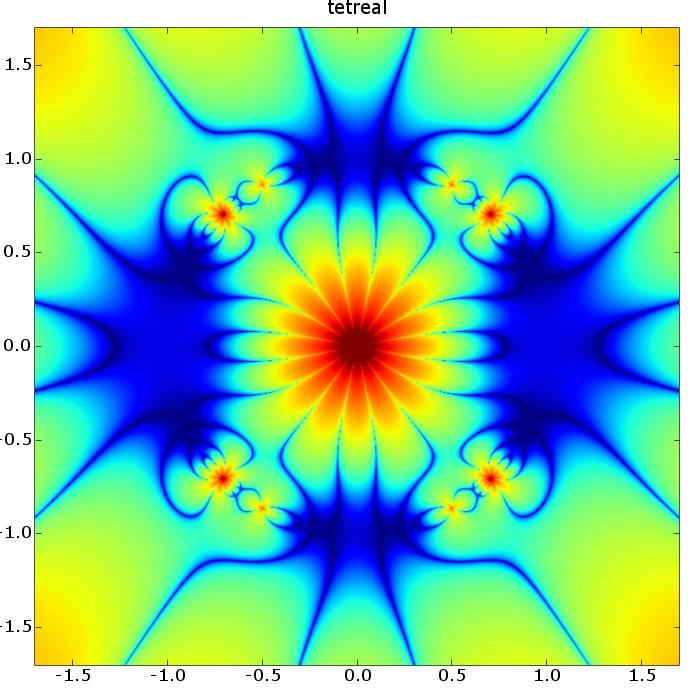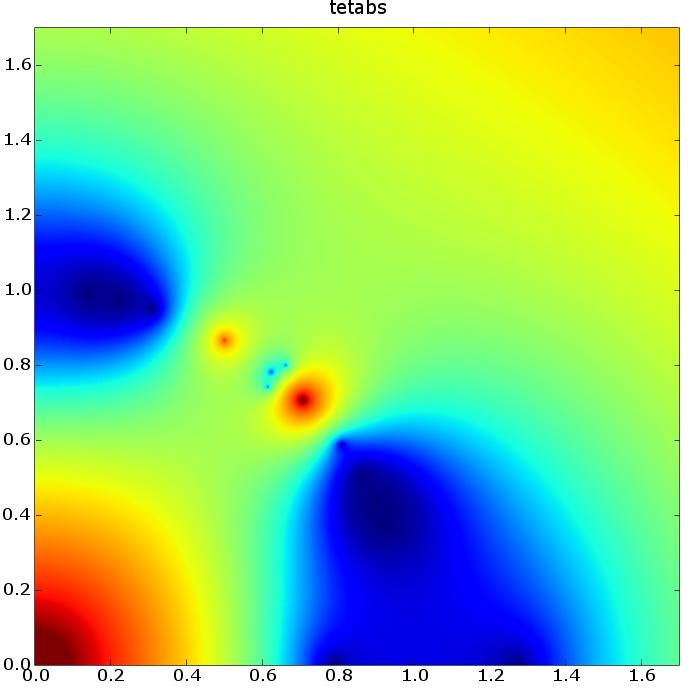(For more information about spin networks, and their relation to quantum gravity, see my quantum gravity web page.)

# Plots of q-deformed tets

The following is a plot of the SUq(2) tet(4,4,4,4,4,4) spin network as a function of the complex deformation parameter q. Analytically, this works out to f(q)/g(q), where

f(q) = (q4-q2+1) (q4+q3+q2+q+1) (q4-q3+q2-q+1) (q6+q5+q4+q3+q2+q+1) (q6-q5+q4-q3+q2-q+1) (q20-q18-q14-q12+q10-q8-q6-q2+1)

and

g(q) = q8 (q4+q2+1)2 (q4+1)5.

This is complex valued, so first a plot of the real part:More precisely, this is a plot of adjust(Re(tet)), where the adjust function is defined by adjust(x) = log(log(abs(x)+1)+1). The double log is because the values of the tet vary wildly. In the image, blue indicates Re(tet) near zero, and red indicates Re(tet) extremely large in absolute value.

And here is adjust(|tet|), where |-| is the complex norm:Here we zoom in on the first quadrant of the above two images. If you make your window wide enough, you can see them side-by-side.These images were produced using python, matplotlib and the spinnet library developed by Igor Khavkine and Dan Christensen.